Next: About this document ... Up: lab_template Previous: lab_template

Subsections

# Cylindrical and Spherical Coordinates

## Background

Defining surfaces with rectangular coordinates often times becomes more complicated than necessary. A change in coordinates can simplify things. The easiest examples are a sphere and a cylinder.
> with(plots):
> f1:=x^2+y^2+z^2=49;
> g1:=rho=7;
> implicitplot3d(f1,x=-7..7,y=-7..7,z=-7..7,axes=boxed,scaling=comstrained);
> implicitplot3d(g1,rho=0..7.5,theta=0..2*Pi,phi=0..Pi,coords=spherical,
numpoints=5000,axes=boxed);
> f2:=x^2+y^2=49;
> h2:=r=7;
> implicitplot3d(f2,x=-7..7,y=-7..7,z=-8..8,axes=boxed);
> implicitplot3d(h2,r=0..7.5,theta=0..2*Pi,z=-8..8,coords=cylindrical,
numpoints=3000,axes=boxed);

To change to cylindrical coordinates from rectangular coordinates use the conversion:
Where
is the radius in the x-y plane and
is the angle in the x-y plane. To change to spherical coordinates from rectangular coordinates use the conversion:
Whereis the angle in the x-y plane;
is the radius from the origin in any direction; and
is the angle in the x-z plane. As an example, the equation of an ellipsoid in rectangular coordinates is
> f3:=x^2/23+y^2/23+z^2/122=1;
>implicitplot3d(f3,x=-5..5,y=-5..5,z=-12..12,scaling=constrained,axes=boxed);

Changing to sherical coordinates:
> g3:=simplify(subs({x=rho*sin(phi)*cos(theta),y=rho*sin(phi)*sin(theta),
z=rho*cos(phi)},f3));
> implicitplot3d(g3,rho=0..12,theta=0..2*Pi,phi=0..Pi,coords=spherical,axes=boxed,
scaling=constrained,numpoints=2000);


## Exercises

1. Given the rectangular equation for a hyperboloid of one sheet:A)
Graph the equation using the domain values of,and the range values.
B)
Write the equation in spherical coordinates and then graph the equation.
C)
Write the equation in cylindrical coordinates and graph it.
D)
Looking at the three equations, which coordinates appears to give the simplest equation?
2. A sphere centered at the origin (as seen in the background) is a very simple equation in spherical coordinates. Now let's look at a sphere moved away from the origin.
A)
Graph the equation using the domain values
,and the range values
.
B)
Write the equation in spherical coordinates and graph it. (You may want to use axes=normal instead of boxed)
C)
Write the equation in cylindrical coordinates and graph it. (You may want to use axes=normal instead of boxed)
D)
Looking at the three equations, which coordinates appear to give the simplest equation?
E)
Looking at the rectangular domain values, a hemisphere can be graphed two ways. Changing the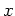values to(or). The second way would be to change the y values to. Graph a hemisphere four ways:
i.
In spherical coordinates change thevalues.
ii.
In spherical coordinates change thevalues.
iii.
In cylindrical coordinates change thevalues.
iv.
In cylindrical coordinates change the
values.Next: About this document ... Up: lab_template Previous: lab_template
Jane E Bouchard
2006-09-27